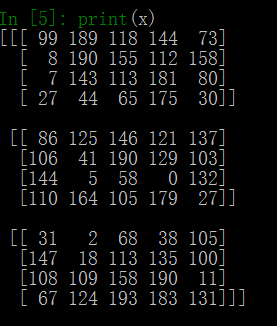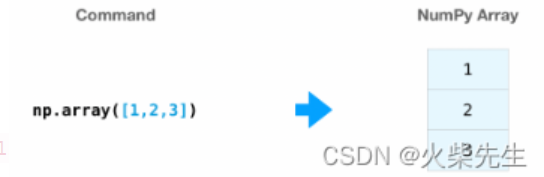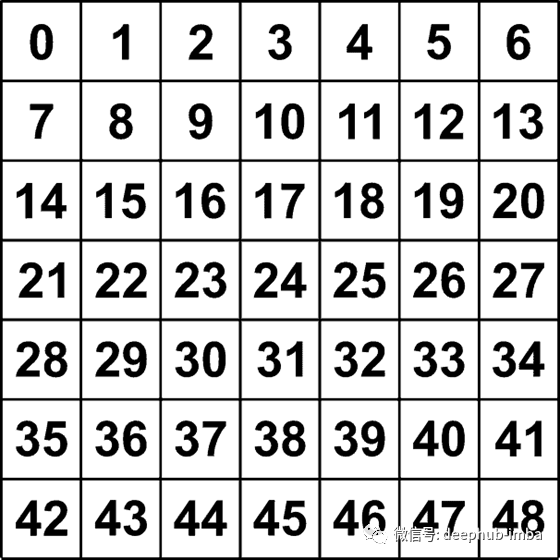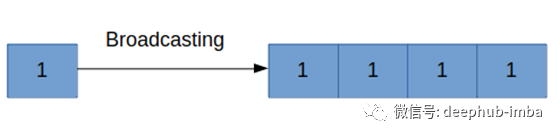# Numpy：高维数组（矩阵）

+关注继续查看

numpy库为python提供了很多方便的数学计算方法，尤其是提供了数组，极大方便了使用python进行矩阵运算，使其在机器学习和深度学习中得到有效利用，本文详细介绍一下高维矩阵问题。

## 正文：

import numpy as np

a=np.arange(10)
print(a)
print(a[0:9])  # 包头不包尾
print(a[3:6])
print(a[:5])  # :前面不写就是从下标为0开始
print(a[5:])  # :后面不写就是一直到最后一个元素
print(a[:])   # :前后都不写就是从头到尾

print('---------------')
'''

b[]内用逗号将各维分开，分别代表第1,2,3...维元素

'''
b= np.mat(np.arange(20).reshape(4,5))
print(b)
print(b[1:3,2:5])   # 先取第一维中下标为1,2的2部分，再取第二维中下标为2,3,4的3部分
print(b[:2,2:])     # 同理，前面不写从头开始，后面不写一直到末尾
print(b[:2,3])      # 当然，也可以在某维度上只取一行

print('-----------------')
c= np.arange(60).reshape(3,4,5)
print(c)
print(c[:2,2:4,1:4])  # 从外向内一层一层的，不改变矩阵维度

print('-------------------')
d= np.arange(240).reshape(3,4,5,4)
print(d)
print(d[:2,1:3,2:5,1:3])Python 数学运算库Numpy入门基础（一）创建数组
Python 数学运算库Numpy入门基础（一）创建数组
20 0numpy数组中冒号[:,:,0]与[...,0]的区别
x[:,:,0] 意思是对数组x切片，可以想象成一个正方体数据，每次切下一个面的数据。第二维取0则得出来[3,4]大小的数组
30 0Numpy中数组和矩阵操作的数学函数
Numpy 是一个强大的 Python 计算库。它提供了广泛的数学函数，可以对数组和矩阵执行各种操作。本文中将整理一些基本和常用的数学操作。
74 094 0Numpy数组——1.数组的创建
Numpy数组——1.数组的创建
94 0Python, Numpy求 list 数组均值，方差，标准差
Python, Numpy求 list 数组均值，方差，标准差
174 0C中处理Python的Numpy数组的代码范例
C中处理Python的Numpy数组的代码范例
15 0【Numpy】数组的创建和常用函数（排序、连接、属性、reshape、索引和切片）
【Numpy】数组的创建和常用函数（排序、连接、属性、reshape、索引和切片）
46 0142 0NumPy中的广播：对不同形状的数组进行操作
NumPy中的广播：对不同形状的数组进行操作
33 0Python学习笔记第三十八天(NumPy 迭代数组)
Python学习笔记第三十八天讲解控制遍历顺序、修改数组中元素的值、使用外部循环、广播迭代的用法。
49 0Python学习笔记第三十四天(NumPy 从数值范围创建数组)
Python学习笔记第三十四天讲解NumPy 从数值范围创建数组、numpy.arange、numpy.linspace、numpy.logspace的用法。
60 0Python学习笔记第三十三天(NumPy 从已有的数组创建数组)
Python学习笔记第三十一天讲解NumPy 从已有的数组创建数组、numpy.asarray、numpy.frombuffer、numpy.fromiter的用法。
37 0
Chin2018

Python 科学计算库 NumPy 快速入门4046714